### Home > A2C > Chapter 3 > Lesson 3.2.2 > Problem3-113

3-113.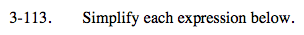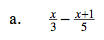Find a common denominator.

$=\frac{5x}{15}-\frac{3(x+1)}{15}$

Simplify the fractions and combine like terms.

$=\frac{5x-3x-3}{15}$

$=\frac{2x-3}{15}$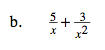See part (a).

$\frac{5x+3}{x^{2}}$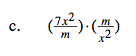Reduce by the Giant One.

$=\frac{7}{1}\cdot\frac{x^{2}}{x^{2}}\cdot\frac{m}{m}$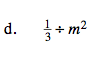Multiply by the reciprocal of m2.